# Second fundamental form

of a surface

The quadratic form in the differentials of the coordinates on the surface which characterizes the local structure of the surface in a neighbourhood of an ordinary point. Let the surface be given by the equation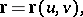whereandare internal coordinates on the surface; let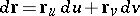be the differential of the position vectoralong a chosen directionof displacement from a pointto a point(see Fig.). Letbe the unit normal vector to the surface at the point(here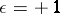if the vector triplet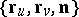has right orientation, and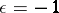in the opposite case). The double principal linear partof the deviationof the pointon the surface from the tangent plane at the pointis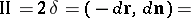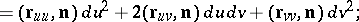it is known as the second fundamental form of the surface.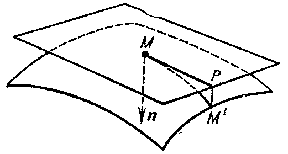Figure: s083700a

The coefficients of the second fundamental form are usually denoted byor, in tensor notation,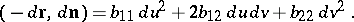The tensoris called the second fundamental tensor of the surface.

See Fundamental forms of a surface for the connection between the second fundamental form and other surface forms.